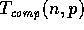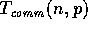Next: Parallel Communications Model Up: Scalable Data Parallel Algorithms Previous: Introduction

# Parallel Algorithm Analysis

A data parallel algorithm can be viewed as a sequence of parallel synchronous steps in which each parallel step consists of an arbitrary number of concurrent primitive data operations. The complexity of a data parallel algorithm can be expressed in terms of two architecture-independent parameters, the parallel time, i.e., the number of parallel steps, and the work, i.e., the total number of operations used by the algorithm . However we concentrate here on evaluating the scalability of our algorithms on two distinct architectures, namely the Connection Machines CM-2 and CM-5. In this case we express the complexity of a parallel algorithm with respect to two measures: the computation complexity\ which is the time spent by a processor on local computations, and the communication complexitywhich is the time spent on interprocessor communication of the overall algorithm. We use the standard sequential model for estimating. However an estimate of the termdepends in general on the data layout and the architecture of the machine under consideration. Our goal is to split the overall computation almost equally among the processors in such a way thatis minimum. We discuss these issues next.Next: Parallel Communications Model Up: Scalable Data Parallel Algorithms Previous: Introduction